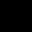# The perimeter of a semi circle is 144 cm then the radius is?

## One Answer on this Question

1.Admin says:

Perimeter of semi circular = πr+2r

Where r is radius , π = 22/7

πr+2r= 144 (given)

(22/7)r +2r = 144

22r+14r = 144*7 (multiply both sides by 7)

36r = 144*7

r= 144*7/36 = 28